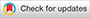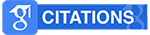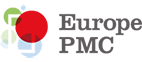# Studi Eksperimen Bandul dalam Menentukan Percepatan Gravitasi Bumi dan Memahami Konsep Gerak Harmonik Sederhana## Abstract

This research is a pendulum experimental study in determining the acceleration of Earth's gravity and understanding the concept of simple harmonic motion. This research was conducted at the Physics Laboratory of the University of Muhammadiyah Lamongan. This study aims to examine the benefits of conducting pendulum experiments with the simple pendulum swing method and in determining the acceleration of Earth's gravity, the period of oscillation and concepts in simple harmonic motion with variations in the mass of the pendulum, namely 150 grams, 75 grams and 35 grams. The results of the analysis of this study obtained the acceleration of Earth's gravity 9.79 m/s where the mass and length of the pendulum do not affect the value of gravity. While in simple harmonic motion in the form of pendulum oscillation is influenced by the length of the string. The pendulum oscillation will experience energy dissipation in maintaining its equilibrium position due to the gravity of the earth.

Keywords:
Earth's Gravity Acceleration; Pendulum Oscillation; Simple Harmonic Motion; Oscillation Period

Citation Metrics: